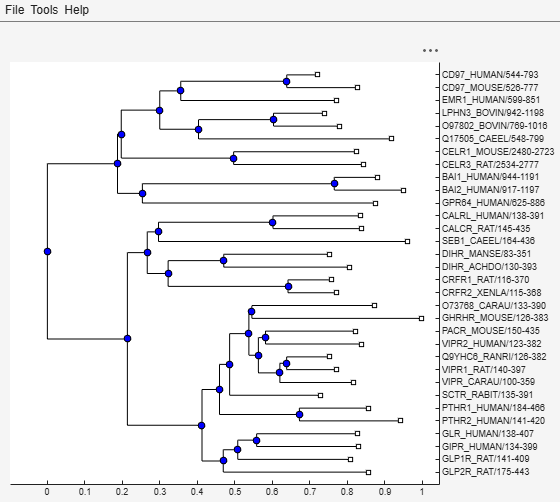# seqneighjoin

Construct phylogenetic tree using neighbor-joining method

## Syntax

```PhyloTree = seqneighjoin(Distances) PhyloTree = seqneighjoin(Distances, Method) PhyloTree = seqneighjoin(Distances, Method, Names) PhyloTree = seqneighjoin(..., 'Reroot', RerootValue) ```

## Input Arguments

 `Distances` Matrix or vector containing biological distances between pairs of sequences, such as returned by the `seqpdist` function. `Method` Character vector or string specifying a method to compute the distances between nodes. Choices are `'equivar'` (default) or `'firstorder'`. `Names` Either of the following:Vector of structures with the fields `Header` and `Name`Cell array of character vectors or string vectorThe number of elements must equal the number of samples used to generate the pairwise distances in `Distances`.

## Description

`PhyloTree = seqneighjoin(Distances)` computes `PhyloTree`, a phylogenetic tree object, from `Distances`, pairwise distances between the species or products, using the neighbor-joining method.

`PhyloTree = seqneighjoin(Distances, Method)` specifies `Method`, a method to compute the distances of the new nodes to all other nodes at every iteration. The general expression to calculate the distances between the new node, `n`, after joining `i` and `j` and all other nodes (`k`), is given by

`D(n,k) = a*D(i,k) + (1-a)*D(j,k) - a*D(n,i) - (1-a)*D(n,j)`

This expression is guaranteed to find the correct tree with additive data (minimum variance reduction).

Choices for `Method` are:

MethodDescription
`equivar` (default)Assumes equal variance and independence of evolutionary distance estimates (a = 1/2), such as in the original neighbor-joining algorithm by Saitou and Nei, JMBE (1987) or as in Studier and Keppler, JMBE (1988).
`firstorder`Assumes a first-order model of the variances and covariances of evolutionary distance estimates, with `'a'` being adjusted at every iteration to a value between `0` and `1`, such as in Gascuel, JMBE (1997).

`PhyloTree = seqneighjoin(Distances, Method, Names)` passes `Names`, a list of names (such as species or products), to label the leaf nodes in the phylogenetic tree object.

``` PhyloTree = seqneighjoin(..., 'Reroot', RerootValue)``` specifies whether to reroot `PhyloTree`. Choices are `true` (default) or `false`. When `RerootValue` is `false`, `seqneighjoin` excludes rerooting the resulting tree, which is useful for observing the original linkage order followed by the algorithm. By default `seqneighjoin` reroots the resulting tree using the midpoint method.

## Examples

collapse all

Create an array of structures representing a multiple alignment of amino acids:

`seqs = fastaread('pf00002.fa');`

Measure the Jukes-Cantor pairwise distances between sequences.

`distances = seqpdist(seqs,'method','jukes-cantor','indels','pair');`

Use the output argument `distances`, a vector containing biological distances between each pair of sequences, as an input argument to `seqneighjoin`.

Build the phylogenetic tree for the multiple sequence alignment using the neighbor-joining algorithm. Specify the method to compute the distances of the new nodes to all other nodes.

`phylotree = seqneighjoin(distances,'equivar',seqs)`
``` Phylogenetic tree object with 32 leaves (31 branches) ```

View the phylogenetic tree:

`view(phylotree)`## References

 Saitou, N., and Nei, M. (1987). The neighbor-joining method: A new method for reconstructing phylogenetic trees. Molecular Biology and Evolution 4(4), 406–425.

 Gascuel, O. (1997). BIONJ: An improved version of the NJ algorithm based on a simple model of sequence data. Molecular Biology and Evolution 14 685–695.

 Studier, J.A., Keppler, K.J. (1988). A note on the neighbor-joining algorithm of Saitou and Nei. Molecular Biology and Evolution 5(6) 729–731.

## Version History

Introduced before R2006a